# Kite

A kite is a quadrilateral with exactly two pairs of adjacent congruent sides.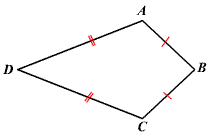This definition excludes squares and rhombi which have all $4$ side congruent.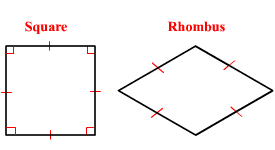### Diagonals:

The longer diagonal of a kite is called the main diagonal and the shorter one is called the cross diagonal. The main diagonal of a kite is the perpendicular bisector of the cross diagonal.

That is, here the diagonal $\overline{\mathit{\text{B}}\mathit{\text{D}}}$ perpendicularly bisects the diagonal $\overline{\mathit{\text{A}}\mathit{\text{C}}}$.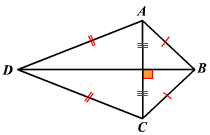Example 1: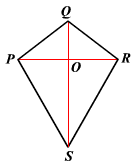In kite $\mathit{\text{P}}\mathit{\text{Q}}\mathit{\text{R}}\mathit{\text{S}}$, $\mathit{\text{Q}}\mathit{\text{S}}$ is the main diagonal. If $\mathit{\text{P}}\mathit{\text{R}}=10$ units and $\mathit{\text{Q}}\mathit{\text{O}}=4$ units what is the length $\mathit{\text{P}}\mathit{\text{Q}}$?

Here, $\mathit{\text{Q}}\mathit{\text{S}}$ is the perpendicular bisector of $\mathit{\text{P}}\mathit{\text{R}}$. Then, $\mathit{\text{m}}\angle \mathit{\text{Q}}\mathit{\text{O}}\mathit{\text{P}}=90°$ and $\mathit{\text{P}}\mathit{\text{O}}=\frac{1}{2}\mathit{\text{P}}\mathit{\text{R}}=\mathit{\text{R}}\mathit{\text{O}}$.

$\mathit{\text{P}}\mathit{\text{O}}=\frac{1}{2}\left(10\right)$

$\text{\hspace{0.17em}}\text{\hspace{0.17em}}\text{\hspace{0.17em}}\text{\hspace{0.17em}}\text{\hspace{0.17em}}\text{\hspace{0.17em}}\text{\hspace{0.17em}}\text{\hspace{0.17em}}=5$

$\Delta \mathit{\text{P}}\mathit{\text{O}}\mathit{\text{Q}}$ is a right triangle, $\mathit{\text{P}}\mathit{\text{O}}=5$ units, $\mathit{\text{Q}}\mathit{\text{O}}=4$ units. Use the Pythagorean Theorem to find the length of the hypotenuse.

$\mathit{\text{P}}\mathit{\text{Q}}=\sqrt{{\left(\mathit{\text{P}}\mathit{\text{O}}\right)}^{2}+{\left(\mathit{\text{Q}}\mathit{\text{O}}\right)}^{2}}$

$\text{\hspace{0.17em}}\text{\hspace{0.17em}}\text{\hspace{0.17em}}\text{\hspace{0.17em}}\text{\hspace{0.17em}}\text{\hspace{0.17em}}\text{\hspace{0.17em}}\text{\hspace{0.17em}}\text{\hspace{0.17em}}=\sqrt{{5}^{2}+{4}^{2}}$

$\text{\hspace{0.17em}}\text{\hspace{0.17em}}\text{\hspace{0.17em}}\text{\hspace{0.17em}}\text{\hspace{0.17em}}\text{\hspace{0.17em}}\text{\hspace{0.17em}}\text{\hspace{0.17em}}\text{\hspace{0.17em}}=\sqrt{25+16}$

$\text{\hspace{0.17em}}\text{\hspace{0.17em}}\text{\hspace{0.17em}}\text{\hspace{0.17em}}\text{\hspace{0.17em}}\text{\hspace{0.17em}}\text{\hspace{0.17em}}\text{\hspace{0.17em}}\text{\hspace{0.17em}}=\sqrt{41}$

The opposite angles at the ends of the cross diagonal are congruent.

That is, $\angle \mathit{\text{B}}\mathit{\text{A}}\mathit{\text{D}}\cong \angle \mathit{\text{B}}\mathit{\text{C}}\mathit{\text{D}}$.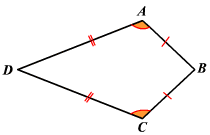### Area:

The area of a kite is half the product of the lengths of the diagonals.

That is, if the lengths of the diagonals of a kite are ${\mathrm{d1}}_{}$ and ${\mathrm{d2}}_{}$ respectively, then area $\mathit{\text{A}}$ of the kite is given by the formula:

$\mathit{\text{A}}=\frac{1}{2}{\mathrm{d1}}_{}\cdot {\mathrm{d2}}_{}$

Example 2:

In kite $\mathit{\text{L}}\mathit{\text{M}}\mathit{\text{N}}\mathit{\text{O}}$, the length of the main diagonal $\mathit{\text{M}}\mathit{\text{O}}$ is $12$ units and that of the cross diagonal $\mathit{\text{L}}\mathit{\text{N}}$ is $5$ units. What is the area of the kite $\mathit{\text{L}}\mathit{\text{M}}\mathit{\text{N}}\mathit{\text{O}}$?

$\mathit{\text{A}}=\frac{1}{2}\left(\mathit{\text{M}}\mathit{\text{O}}\right)\left(\mathit{\text{L}}\mathit{\text{N}}\right)$

$\text{\hspace{0.17em}}\text{\hspace{0.17em}}\text{\hspace{0.17em}}\text{\hspace{0.17em}}=\frac{1}{2}\left(12\right)\left(5\right)$

$\text{\hspace{0.17em}}\text{\hspace{0.17em}}\text{\hspace{0.17em}}\text{\hspace{0.17em}}=\frac{1}{2}\left(60\right)$

$\text{\hspace{0.17em}}\text{\hspace{0.17em}}\text{\hspace{0.17em}}\text{\hspace{0.17em}}=30$

Therefore, the area of the kite $\mathit{\text{L}}\mathit{\text{M}}\mathit{\text{N}}\mathit{\text{O}}$ is $30$ square units.